Ontario Tech acknowledges the lands and people of the Mississaugas of Scugog Island First Nation.

We are thankful to be welcome on these lands in friendship. The lands we are situated on are covered by the Williams Treaties and are the traditional territory of the Mississaugas, a branch of the greater Anishinaabeg Nation, including Algonquin, Ojibway, Odawa and Pottawatomi. These lands remain home to many Indigenous nations and peoples.

We acknowledge this land out of respect for the Indigenous nations who have cared for Turtle Island, also called North America, from before the arrival of settler peoples until this day. Most importantly, we acknowledge that the history of these lands has been tainted by poor treatment and a lack of friendship with the First Nations who call them home.

This history is something we are all affected by because we are all treaty people in Canada. We all have a shared history to reflect on, and each of us is affected by this history in different ways. Our past defines our present, but if we move forward as friends and allies, then it does not have to define our future.

# Derivative Rules

In calculus, a derivative can be thought of as an instantaneous rate of change; that is, how much a quantity is changing at a given point. Let’s take a closer look at how we can differentiate a function easily by the use of some helpful rules.

## Understanding the Derivative

Differentiation is a method to compute the rate at which a dependent variable y changes with respect to the change in the independent variable x. This rate of change is called the derivative of y with respect to x. There are many different notations to denote “take the derivative of.” The relationship between y and x is usually denoted by f(x) and its derivative is usually denoted as f‘(x) or y’ or dy/dx. The definition of the derivative is given by: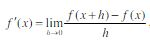which is a lengthy procedure used to evaluate the derivative of a function. Thankfully, easier methods have been developed to help evaluate derivatives more quickly.

### The Power Rule

If n is any real number, then  d (xn) = nxn-1
dx
Also, remember that the derivatives of a constant is zero:  (c) = 0.
dx

Note that the sum and difference rule states: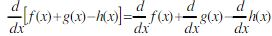(Just simply apply the power rule to each term in the function separately).

Example: Find the derivative of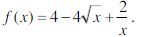Solution:

First, rewrite the function so that all variables of x have an exponent in the numerator: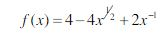Now, apply the power rule to the function: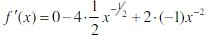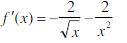### The Product Rule

We already know how to find the derivative of a sum or difference of functions, but what about the product of two functions? The product of two functions is when two functions are being multiplied together. If f and g are both differentiable, then the product rule states: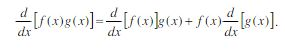Example: Find the derivative of h(x) = (3x + 1)(8x4 +5x).

Solution:

Using the above formula, let f(x) = (3x+1) and let g(x) = (8x4 + 5x).

Now, find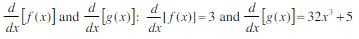Lastly, apply the product rule using the above formula: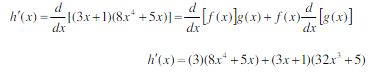### The Quotient Rule

Now, let's take a look at the quotient of functions (when two functions are being divided by each other). If f and g are both differentiable, then the quotient rule states: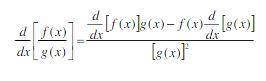Example: Find the derivative of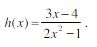Solution:

Using the above formule, let f(x) = (3x-4) and let g(x) = (2x2-1).

Now, find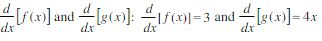Lastly, apply the quotient rule using the above formula: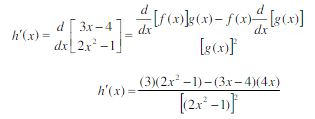### The Chain Rule

If f and g are both differentiable and F is the composite function defined by F(x) = f(g(x)), then F is differentiable and the chain rule can be applied to F ', which is given by  F '(x) = f '(g(x))g '(x)

Example: Find the derivative of F(x) = (3x4 + 2x2 -7)5.

Solution:

Using the above formula, let g(x) = (3x4 + 2x- 7) and let f(x) = (x)5.

Now, find f '(x) and '(x):  f '(x) = 5x4 and '(x) = 12x3 + 4x - 0

So, if f '(x) = 5x4, then the composite function f '(g(x)) = 5(3x4 + 2x2 - 7)4

Lastly, apply the chain rule using the above formula: '(x) = f '(g(x))g '(x)

'(x)  = 5(3x4 + 2x﻿2﻿ -7)﻿4﻿ (12x﻿3﻿ + 4x﻿)